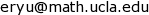## Machine Learning Theory, Spring 2024

This is the public course website for Machine Learning Theory, Spring 2024.

## Announcements

• In 2024, the Mathematics department will offer two new graduate-level courses. In Spring 2024, I will teach Machine Learning Theory. In Fall 2024, Modern Computational Mathematics will be offered, but not by me. These two courses will serve as the "quals courses" for the new computational mathematics quals.
• Previously, the SNU Math Ph.D. qualifying exams (quals) had three subject areas: analysis, algebra, and geometry/topology. The new computational mathematics qual will serve as a 4th option, and Ph.D. students must pass 3 out of the 4 subjects. The comp math qual exam will be first administered in January 2025. Further details of the new quals will soon be determined and announced by the department.

## Course Description

This course will cover classical machine learning theory (but not necessarily the modern "deep learning theory") and some optimization theory. Topics will include: PAC learning, VC dimension, Rademacher complexity, kernel methods and RKHS, gradient descent, Newton's method, stochastic gradient descent, and continuous-time models of gradient descent.

## Course Information

### Instructor

Ernest K. Ryu, 27-205,### Exams

This class will have in-person midterm and final exams.

### Prerequisites

Good knowledge of the following subjects is required.

• Differential calculus and linear algebra.
• Undergraduate analysis: $\epsilon$-$\delta$ argument, compact sets, uniform convergence.
• Basic ODEs: Initial value problem.
• Probability theory: Conditional expectation, central limit theorem, and law of large numbers.
• Mathematical maturity: The focus of this course will be on rigorous mathematical proofs, as opposed to derivations or implementations.

### Non-Prerequisites

Background knowledge in the following areas is helpful but not necessary.

• This course will have no programming component; we will analyze (implementable) algorithms, but we will not implement them.
• Background in machine learning or deep learning is not required, although prior exposure will help motivate the subject matter.
• Measure-theoretic probability theory is not required, but we will discuss the measure-theoretic considerations in passing.
• Optimization theory (convex or non-convex) is not assumed.